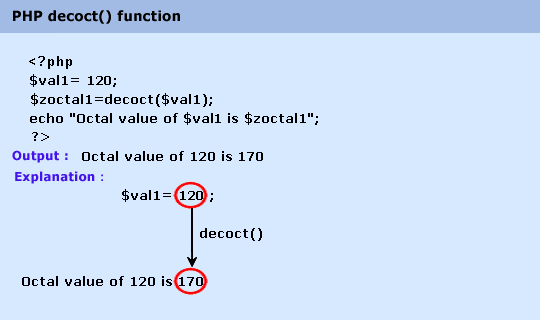# Education is not limited to just classrooms. It can be gained anytime, anywhere... - Ravi Ranjan (M.Tech-NIT)

## PHP : decoct() function

Description

The decoct() function is used to convert a decimal number to an octal number.

Version

(PHP 4 and above)

Syntax

decoct(num)

Parameter

Name

Description

Required /
Optional

Type

num

The decimal value to convert

Required

Integer

Return value

Octal string representation of num.

Value Type : String.

Pictorial PresentationExample :

1. \$val1= 120;

2. \$val2=7;

3. \$val3=9899;

4. \$zoctal1=decoct(\$val1);

5. \$zoctal2=decoct(\$val2);

6. \$zoctal3=decoct(\$val3);

7. echo "Octal value of \$val1 is \$zoctal1
";

8. echo "Octal value of \$val2 is \$zoctal2
";

9. echo "Octal value of \$val3 is \$zoctal3
";

10. ?>

Output :

Octal value of 120 is 170
Octal value of 7 is 7
Octal value of 9899 is 23253